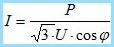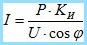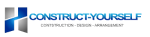Wiring

# Calculation of the cross section current-carrying core of the cable

The main condition for the correct and smooth operation of appliances and equipment is properly designed power supply system. Here it is important to calculate cross sections of current-carrying cable – this should be done in accordance with applicable electrical codes – electrical safety rules Chapter 1.3 seventh edition.

## The main methods of calculation of the cross section of the conductive core of the cable ↑

The main parameters that must be considered when calculating the cross section of the conductive core of the cable are:

• R – power (kW).
• I – nominal current of the network (A).
• U – voltage (B).
• the number of phases.
• the material of the conductor.

To understand how to calculate the cross section of the conductive cable core for domestic purposes, consider the standard two-bedroom apartment.

## The procedure for calculating cross sections ↑

1) it is Necessary to determine the total values of power consumption separately for each room, then these figures add up. For example, the total power in the kitchen is calculated as follows:

• lighting – two light bulbs of 100 watts.

• hood – 100 watt.

• refrigerator – 350 watt.

All of these values should be put – 2х100+100+350+450= 1100 Watts. The same calculations should be performed to the rest rooms.after that, the obtained results are summed and the result is total power consumption. Today, the average this figure is 7.5-8 kW.

2) Choose material gilgames. Usually aluminium or copper.

3) to Determine the voltage and number of phases. In most cases, this is a single-phase network with a voltage of 220 volts. In some homes there is a three-phase network with a voltage of 380 volts. Often, individual houses and private hotels.

It is important to take into account. When calculating the total power needed is always the value obtained is rounded in a big way. If as a result the design becomes an integer, then it is necessary to add 1.0. This is to ensure that the electrical system had a certain margin of safety.Another important point in calculation of total capacity – if you plan in the future purchase of any equipment or appliances (microwave, food processor, dishwasher), their capacity also must be considered.

After the calculation of total power consumed energy you must choose the material from which the cable core. Selection of wire or cable mono to implement special tables, which are available on the Internet and in the literature. In our case, the value of the cable diameter and for aluminum would be 6 mm2. (single phase – 220 V or 4 mm2. – copper vein). When the three-phase connection is used the reduction factor. For example, if the total power consumption is 7.5 kW., the required cable cross-section 1.5 mm2. – copper and 2.5 mm2. – aluminium.### Current-carrying core of the cable load ↑

In this embodiment, the calculations for the basic indicator maximum allowable load (amperage).To calculate the force of the current flowing in the network, you have total power to apportion the voltage – our case is 7500/220 = 34,09 is the load current. Under current regulations, it is customary to use use the following ratio was 1 mm2. the cross-section of current-carrying conductors have 4 A. So it turns out 34,09/4 = of 8.52 mm2. Then, referring to special tables, the selection is made of the cross section of conductors depending on the conductor material, voltage and number of phases.

### The program for the calculation of the cross section current-carrying core of the cable ↑

For those who don’t know how to calculate the cross section current-carrying core of the cable or doubt of the correctness of their calculations, there is a special program with which you can quickly and accurately carry out calculation of the cross section current-carrying core of the cable. It is enough to download on PC (for free), enter the required parameters and get the result.

Content related